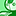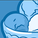# New to Qlik Sense

If you’re new to Qlik Sense, start with this Discussion Board and get up-to-speed quickly.

Announcements
Don't miss the upcoming Q&A with Qlik session on Qlik Application Automation on November 16th! REGISTER NOW
cancel
Showing results for
Did you mean:Partner

## Using different expression when choosing different month

I would like to use formula 1 if Month <10, otherwise use formula 2.

I tried to write my expression like this:

If ( Month < 10,

formula 1,

formula 2)

However,If I select Month = 10 and Month = 9 and Month = 8, the total value always calculated by formula 2,

Total value not formula 2 value + formula 1 value + formula 1 value.

e.g If( Month <10,1,2). When select Month = 10, the value = 1, when select Month = 11, the value =2. However, I select Month = 10 and 11, the value = 2, not equal to 3

1 Solution

Accepted SolutionsSpecialist

A lot of the behavior depends on how your formulas are written and when the aggregation occurs.  One strategy that you can try is to move the month logic to set analysis.

{\$<Month={"<10"}>} and {\$<Month={">=10"}>}, respectively in your expressions.

Then, if the formulas are added together in the expression, the appropriate formula will be used for each month even when selections are made in the month field.Specialist

A lot of the behavior depends on how your formulas are written and when the aggregation occurs.  One strategy that you can try is to move the month logic to set analysis.

{\$<Month={"<10"}>} and {\$<Month={">=10"}>}, respectively in your expressions.

Then, if the formulas are added together in the expression, the appropriate formula will be used for each month even when selections are made in the month field.Tags
Community Browser